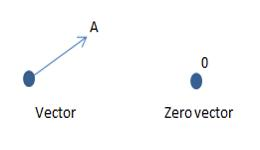# What is Null Vector or Zero Vector

Null vector or zero vector: A vector whose magnitude is zero, is called a null vector or zero vector. It is represented by Ȏ and its starting and end points are the same. The direction of null vector is not known.In mathematics, a vector merely an element of a vector space. A vector space is merely a set with binary operations which satisfy the vector space axioms. It’s a very abstract thing.